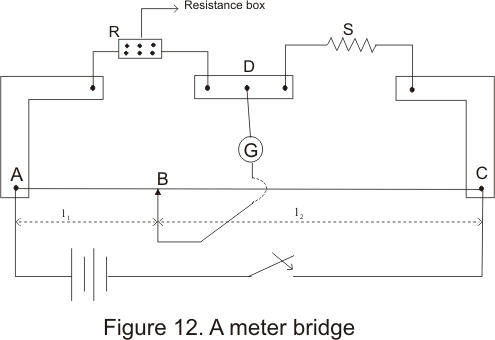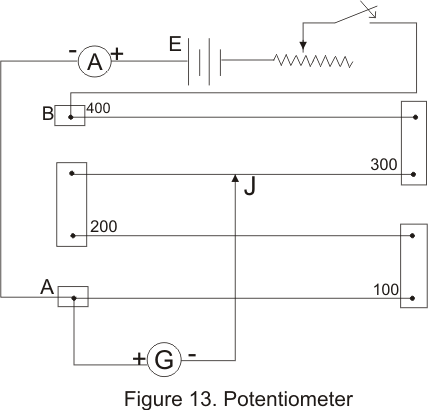# Meterbridge|Potentiometer

## (8) Meterbridge (slide wire bridge)

• Meter bridge is based on the principle of wheatstone bridge and it is used to find the resistance of an unknown conductor or to compare two unknown
resistance
• Figure below shows a schematic diagram of a meter bridge• In above figure AC is a 1m long wire made of maganin or constanan having uniform area of cross-section
• This wire is stretched along a scale one a wooden base
• Ends A and C of the wire are screwed to two L shaped copper strips as shown in figure
• A resistance box R and an unknown resistance S are connected as shown in figure
• One terminal of galvanometer is connected to point D and another terminal is joined to a jockey that can be slided on a bridge wire
• when we adjust the suitable resistance of value R in the resistance box and slide this jockey along the wire then a balance point is obtained sat at point B
• Since the circuit now is the same as that of wheatstone bridge ,so from the condition of balanced wheatstone bridge we have
P/Q=R/S
Here resistance P equals
P=ρl1/A
And Q=ρl2/A
where ρ is the resistivity of the material of the wire and A is the area of cross-section of wire
Now P/Q=(ρl1/A)(A/ρl2)=l1/l2

## (9) Potentiometer

• Potentiometer is an accurate instruments used to compare emf's of a cells,Potential difference between two points of the electric wire
• Potentiometer is based on the principle that potential drop across any portion of th wire of uniform crossection is proportional to the length of that portion of thw wire when a constant current flows through the wire
• Figure below shows the construction of a potentiometer which consists of a number of segments of wire of uniform area of cross-section stretched on a wooden board between two copper strips .Meter scale is fixed parallel to the lenght of the wire• A battery is connected across terminals A and B through a rehestat so that a constant currents flows through the wire
• Potentiometer is provided with a jockey J with the help of which contact can be made at any point on the wire
• Suppose A and ρ are the area of cross-section and resistivity of the material of the wire the resitance
R=ρl/A ----------------------------(i)
where l is the lenght of the wire
• If I is the current flowing through the wire then from Ohm's Law,
V=IR ------------------------------(ii)
Where V is the potential differene across the position of the wire of length l
Thus ,from (i) and (ii)
V=IR=I(ρl/A)=kl
where K=ρI/A
=> V is proportional to l when current I is constant
• K=V/l is also known as potential gradient which is the fall of potential per unit length of wire
• Senstivity of a potentiometer depends on its potential gradient .If the potential gradient of a potentiometer is small then the potentiometer is more sensitive and hence more accurate